﻿ 电光晶体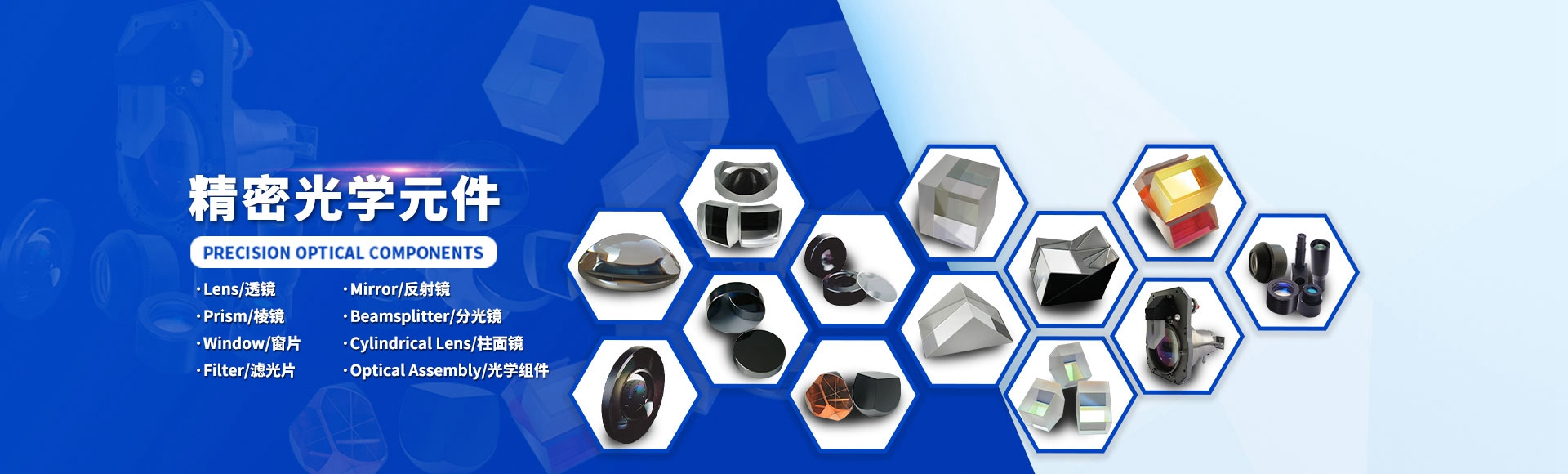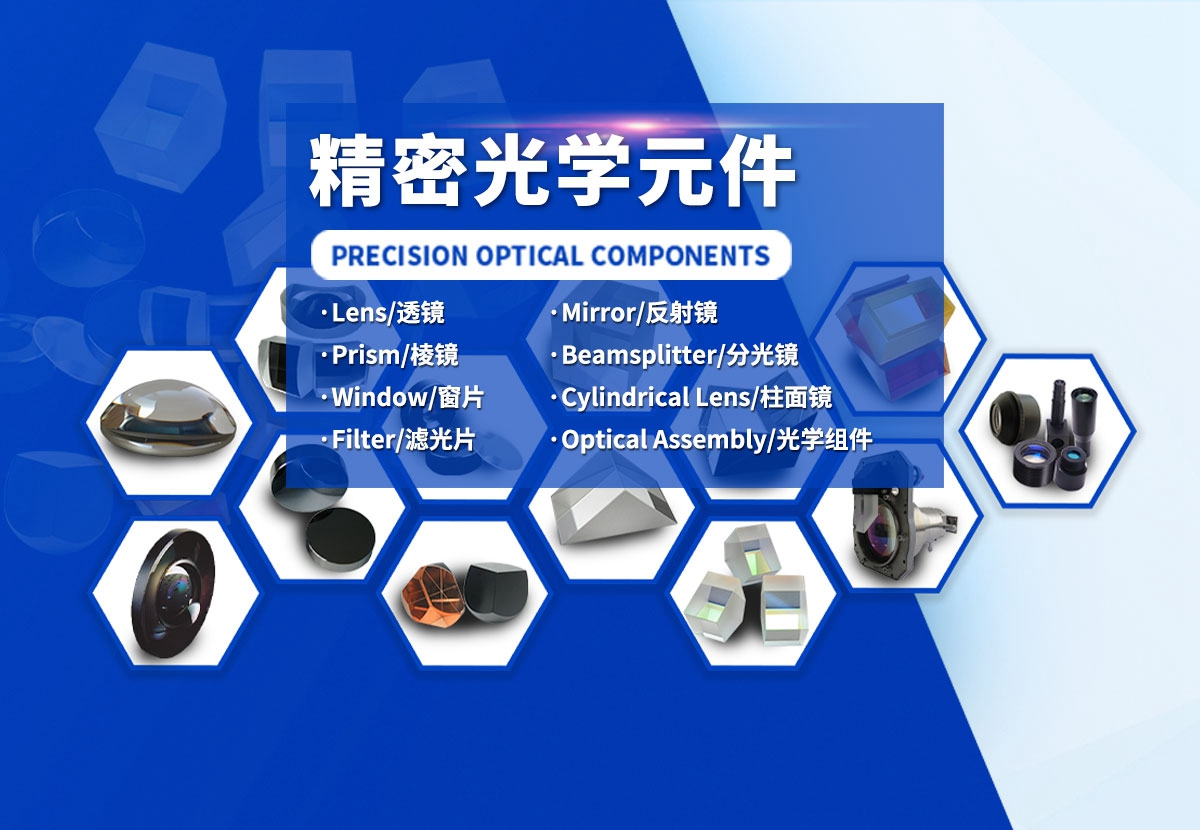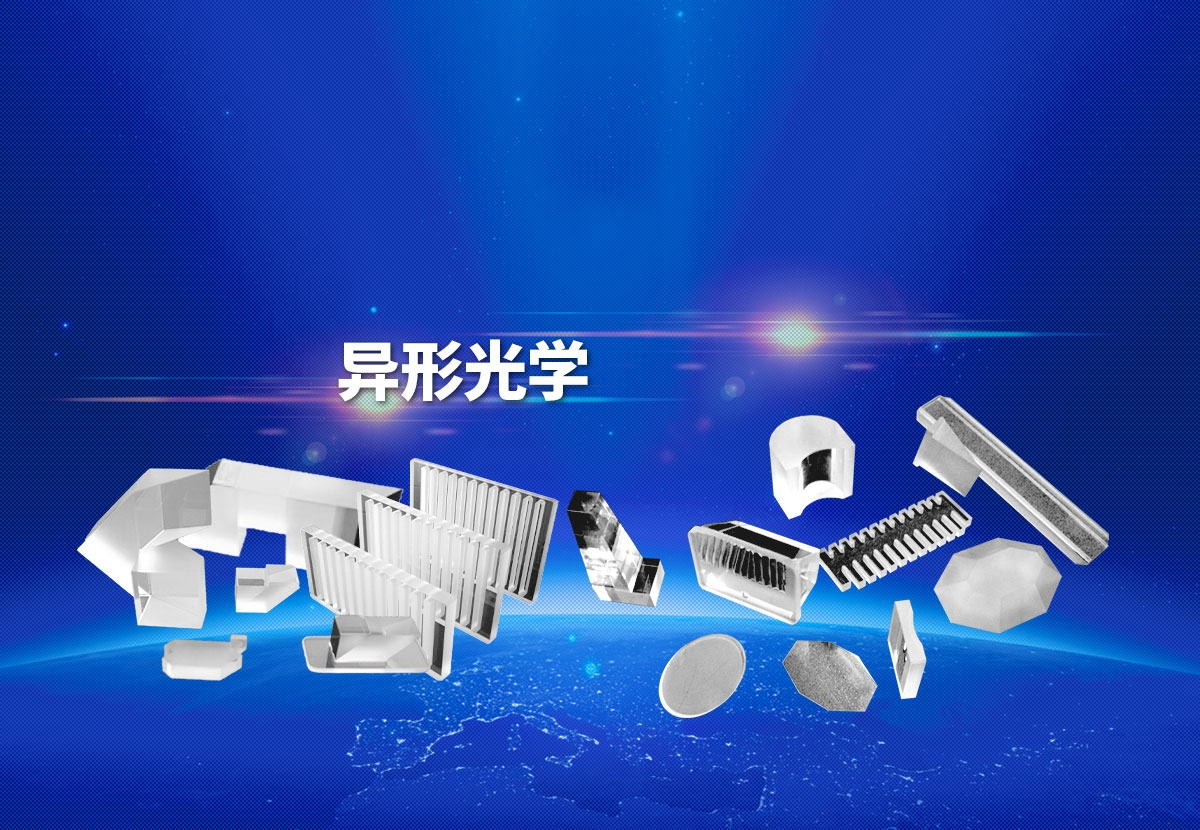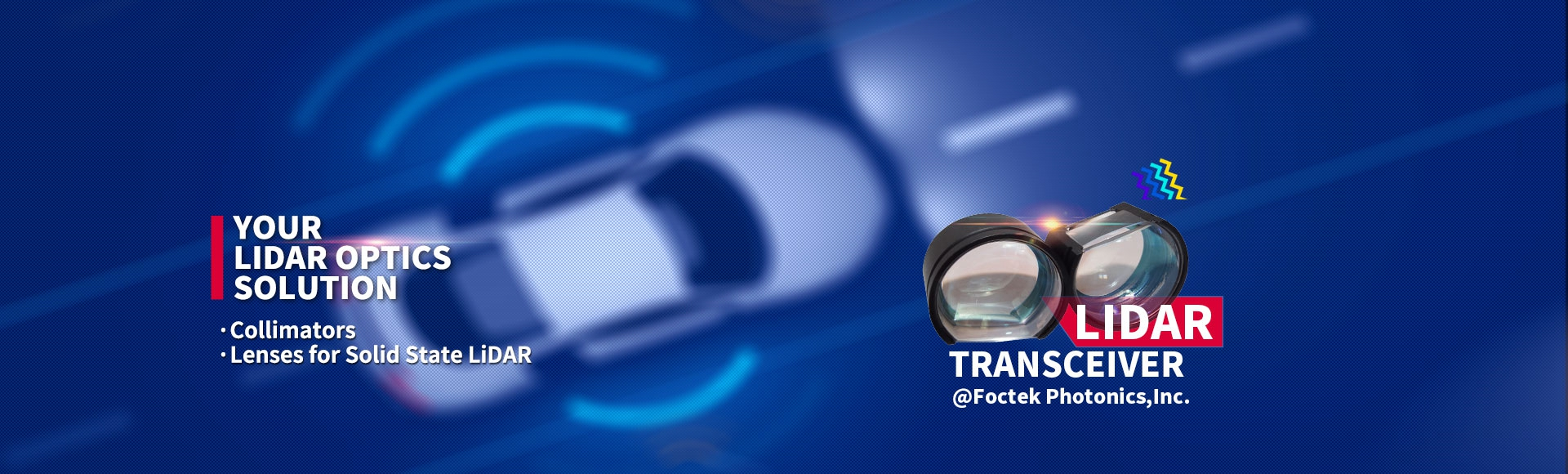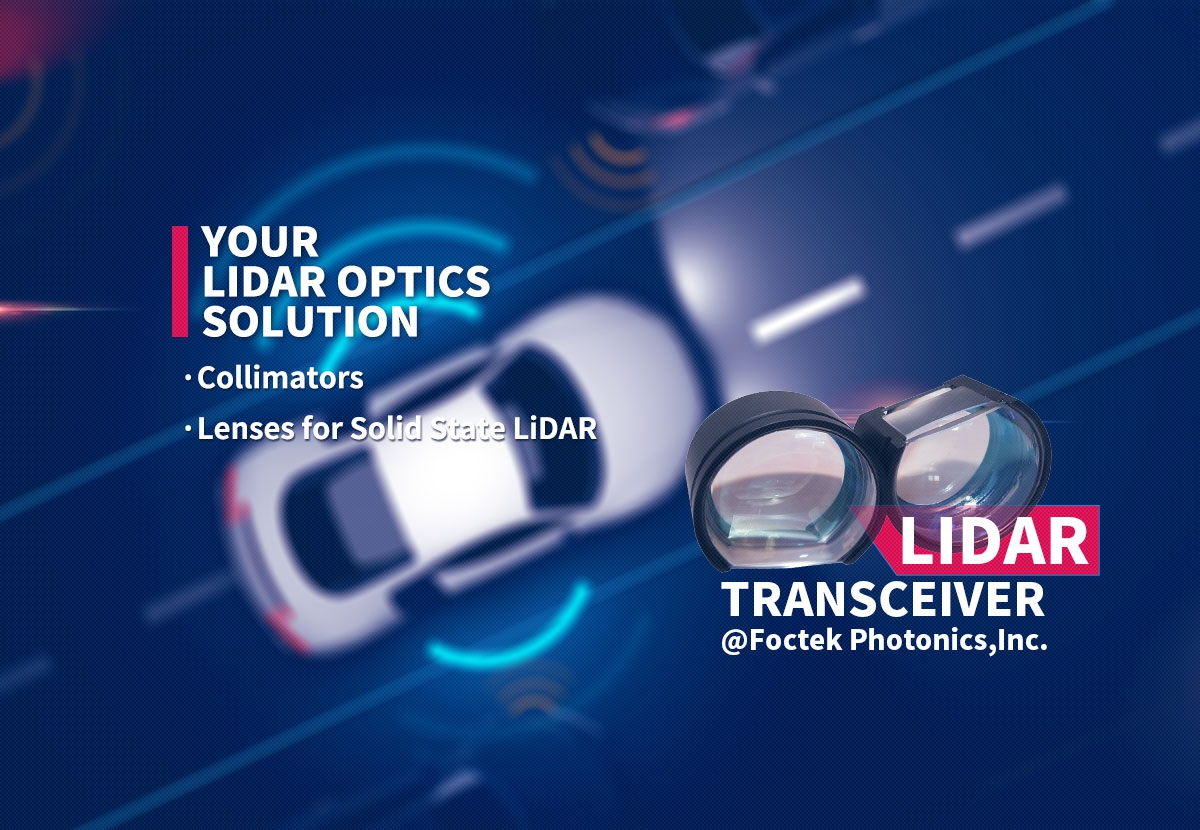主营业务 >> 精密光学事业部 >> 产品介绍 >> 晶体产品 >> 电光晶体

Electro-optic effect is the change in the refractive index resulting from the appliation of a DC or low frequency electric field. A field applied to an ansotropic electro-optic material can modify its refractive indices and thereby its effect on polarized light. The dependence of the refractive index on the applied electric field takes two forms: linear electro-optic effect, and quadratic electro-optic. Electro optics crsyals

can be used for producing controllable optical devices such as Q-switch application.
If a linearly polarized light passes through an E-O crystal, the phase retardation (T) will be induced by An to r = 2πOnL, where L is crystal length, for KD*P，as an example, An=0.5 ∩30F6sE/N，r = πLn3ofFx3E/n. It is clear that the phase of light will change together with electric field (E). This is called electro-optic phase modulation. If two crossed polarizers are placed at input and output ends of E-O crystal separately as shown in (Amplitude Modulator), the output intensity of light will be | = I。sin2(G/2), where l。 is input intensity. That means the intensity or amplitude of light can also be modulated by electric field. This is called amplitude modulation.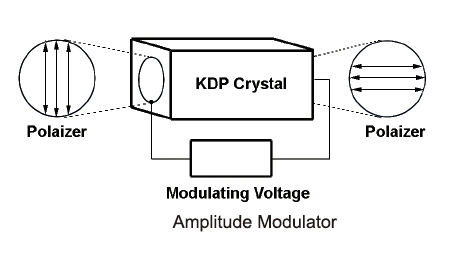There are two kinds of E-O modulations. One is longitudinal E-O modulation if the directions of electric field and light propagation are the same (as shown in Longitudinal Modulation). The KDP isomorphic crystals are normally used in this scheme. If the directions of electric field and light propagation are perpendicular, it is called transverse E-O modulation (see Transverse Modulation). The LiNbO3, MgO:LiNbO3, ZnO:LiNbO3, BBO and KTP crystals are usually employed in this scheme.The half-wave voltage (V) is defined as the voltage which makes T = π, for example, V =2/(2nre3) for
KD*P and V, =λd/(2nr22L) for LiNbO3, where λ is light wavelength and d is the distance between the
electrodes.